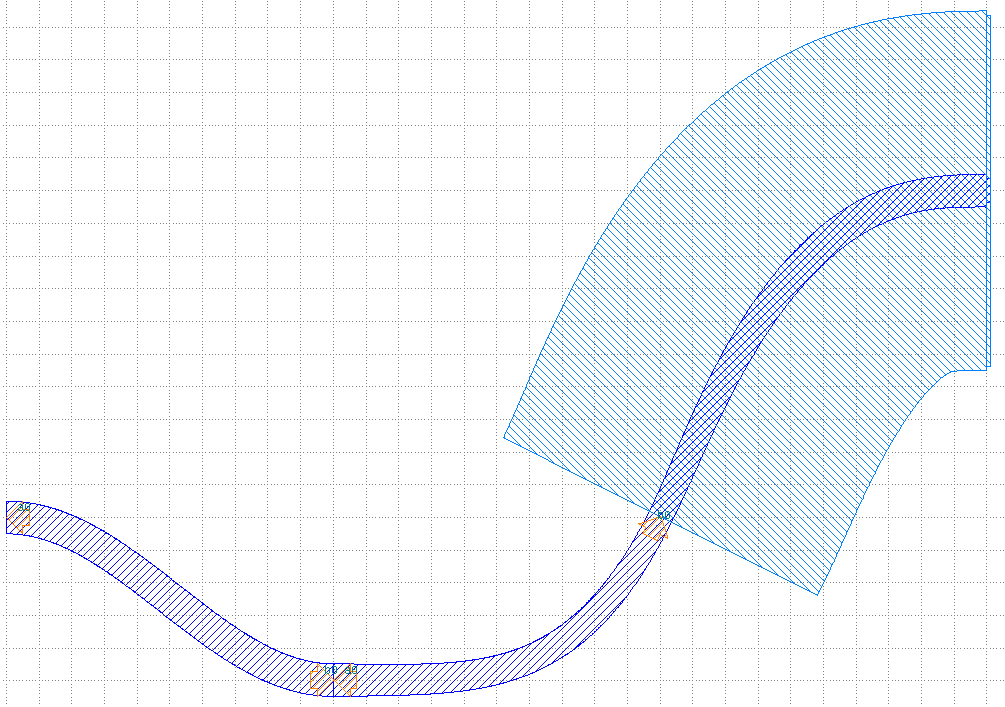Home Forums Nazca Questions and Answers Procedure to create a flexible interconnect type

Viewing 3 posts - 1 through 3 (of 3 total)
• Author
Posts
• #6274

Hello Nazca team & fellow users,

I wanted to create a flexible taper type that gives me a lot of flexibility in tapering and bending.  Specifically I want to define the rate of width tapering with a function, and the progression of a bend offset with a function as well.  This enables a lot of control that’s very useful in designing some passive photonic devices.

I created the function “custom_offset_taper” which I will attach below.  I haven’t tested it rigorously, but so far it does everything I need it to do.  I am wondering if there’s a better way to achieve the same functionality making use of the Nazca library and have better interoperability with other Nazca functions?

Specific issues I have are:

1) It only works with a single layer, not cross-sections

2) If I want to connect a cobra_p2p to the end of the interconnect and match the radius-of-curvature, it’s cumbersome.  I set custom_offset_taper up so it can either return a cell handle, or a dictionary of stats including r.o.c.  I have to call the function twice.  I did try making it return a tuple of (cellname, stats), and then in the next line put cellname.put(), but the .put() command would always fail.

I would value any feedback, as I learn to utilize Nazca more effectively.

Thank you!

Jon

``````import nazca as nd
import nazca.interconnects as IC
import numpy as np

########################################################################################################################
#Define Layers & Cross Sections
########################################################################################################################

nd.clear_layers()

########################################################################################################################
#Define layout functions
########################################################################################################################

#Custom taper with offset & parametric function defined shape.
#in_slope and out_slope can be overridden to set input angle to 0, etc. Default setting 'None' is software determined
#if flat=False, ports can be angled, widths refer to waveguide widths regardless of angle.
#If flat=true, ports point horizontally, width is vertical width.
#returning output stats is optional.  If returned, ".put(..)" needs to be put on a separate line.
#  purpose is so radius of curvature can be returned and used in subsequent interconnects.
def custom_offset_taper(length, offset, width1, width2, layer='rib', label='custom_offset_taper', in_slope=None,
out_slope=None, flength=lambda x: x, fwidth=lambda x: x, foffset= lambda x: x, npoints=500, flat=False, output_stats=False):
frac_pts = np.linspace(0,1,npoints)
lengths = length*flength(frac_pts)
offsets = offset*foffset(frac_pts)
widths = width1 + (width2-width1) * fwidth(frac_pts)
d = lambda x: np.concatenate((x[1:],[x[-1]])) - np.concatenate(([x],x[0:-1])) #Derivative
dydx = d(offsets)/d(lengths)
d2ydx2 = d(dydx)/d(lengths)
if in_slope is not None:
dydx=in_slope
if out_slope is not None:
dydx[-1]=out_slope
angs = np.arctan(dydx)
in_ang = 0 if flat else angs*180/np.pi
out_ang = 0 if flat else angs[-1]*180/np.pi
top_edge_x = lengths - 0.5*widths*(0 if flat else np.sin(angs))
bot_edge_x = lengths + 0.5*widths*(0 if flat else np.sin(angs))
top_edge_y = offsets + 0.5*widths*(1 if flat else np.cos(angs))
bot_edge_y = offsets - 0.5*widths*(1 if flat else np.cos(angs))
top_pts = list(zip(top_edge_x,top_edge_y))
bot_pts = list(zip(bot_edge_x,bot_edge_y))
bot_pts.reverse()
poly_pts = top_pts + bot_pts
roc = np.divide((1 + (dydx)**2)**1.5, d2ydx2, out=np.zeros_like(d2ydx2), where = (d2ydx2!=0)) #radius of curvature
#Above line: Avoid divide by zero: https://stackoverflow.com/a/37977222
argminroc = np.argmin(np.abs(roc))
print(poly_pts)
with nd.Cell(name=label) as cell: #, autobbox=True) as cell:
curve = nd.Polygon(points=poly_pts, layer=layer).put(0)
nd.Pin('a0', width=width1).put(0,0,180+in_ang)
nd.Pin('b0', width=width2).put(length,offset,out_ang)
nd.put_stub()
stats={'in_ang':in_ang, 'out_ang':out_ang,
'in_roc':roc, 'out_roc':roc[-3], #Radius of curvature inaccurate at endpoints of derivative, so this does not use endpoints.  Use a lot of points to increase accuracy of returned values.
'min roc':roc[argminroc], 'w_min_roc':widths[argminroc]}
if output_stats:
#return (cell, stats) #This does not work.  A subsequent command of "cell.put(0)" would fail.
return stats
else:
return cell

def component():
with nd.Cell(name='test') as cell:
bend1 = custom_offset_taper(10,-5,1,1, foffset = lambda x: 0.5*(1+np.sin((x-0.5)*np.pi))).put('a0',0,0,0) #sine bend
bend2_stats = custom_offset_taper(10,5,1,0.75, foffset= lambda x: x**4, label='bend 1', output_stats=True)
bend2 = custom_offset_taper(10,5,1,0.75, foffset= lambda x: x**4, label='bend 1', ).put('a0',bend1.pin['b0']) #nonlinear curvature/offset
stub = nd.strt(length = 0.1, width = 0.75, xs='example').put('a0',30,10,0)
ic1.cobra_p2p(pin1=bend2.pin['b0'], pin2=stub.pin['a0'], width1=0.75,
return cell

########################################################################################################################
nd.clear_layout()
cell = component()
nd.export_gds(topcells=cell, filename='example.gds', clear=True)``````#6280

Dear Jon,

Would the free_form_curves approach work for your case?

Ronald

#6286

Hi Ronald,

I had a chance to try replacing all my custom curves with Vipers.  It worked in some cases, but there’s a case for which it doesn’t apply:

My code has a flag called “flat” which effectively makes the inputs and outputs horizontal.  I had drawn a taper which can be described as follows: Inputs and outputs are different widths. One waveguide edge is a straight line.  The other waveguide edge is a parabolic curve.

Would there be a way to render this using the standard Nazca library of functions?

Also: Do ports store curvature information, so that if I do an IC.Interconnect, does it automatically match the curvature of the ports it is connected to?

Thank you!

Jon

Viewing 3 posts - 1 through 3 (of 3 total)
• You must be logged in to reply to this topic.